In order to obtain high-precision photometric measurements of the magnetic regions upon the solar disk, we have to make a careful instrumental calibration of acquired data. In particular, this can only be done through a precise calibration of the answer of the CCD detector to a flat-field.
For PSPT observations, as well as for other whole-disk observations of the
Sun, the calibration for flat-field answer is made through the so-called  “shifted-image” method (Kuhn, Lin e Loranz, 1991). As for the images acquired through the PSPT in Rome, this method is applied through a procedure developed in IDL by the same authors.
As a general rule, this method enables to detect variations of the relative gain upon CCD with an accuracy higher than 10-4. This method involves the use of moved images of the Sun upon the detector in order to re-create the description of differences of gain between the various elements of the detector. A series of 16 shifted solar images are used (each one being the sum of 10 exposures, in our case), individually obtained through the translation from the center of the solar disk (shifting between 5 and 150 pixel), a single centered image (summing up 25 exposures) corresponding to the reference image, and a dark image (summing up, once again, 25 exposures), acquired with closed shutter.
During the acquisition of all necessary images, the optical and acquisition system is not changed. This enables a simultaneous evaluation of both dishomogeneous electronic gain of the elements of the CCD sensor and of possible effects of dishomogeneous lighting upon the sensor due to the telescope’s optical system.
The correct application of the method involves the observance of certain primary hypotheses concerning both the detector and the observed source:
1) the CCD detector must give a linear answer;

2) the calculated gain (namely, the spatial non-uniformity of the answer on the part of the overall detection system), is not only referred to the detector’s anwer, but also to other variations contributing by multiplication to the dishomogeneity of the optical sensitivity of pixels;

3) the shifted images of the source must be just alike one another.

The latter hypothesis, only approximatively realized, involves strong constraints upon the accuracy of the obtained correction. The logic beneath the algorithm can be easily understood. If you consider 3 images, one fixed and the others obtained by shifting by 1 pixel – both in the vertical and horizontal direction – the initial image.
If the image shows only a shifting effect in the next representations, each couple of corresponding pixels (both horizontally and vertically) is enlightened by the same flow: it is therefore possible to calculate the gain ratio of adjoining pixels. Analitically, and more generally, this can be described as follows: consider N identical images shifted among them in a way we know. The answer of the pixel detected by the vector upon the detectors’ plane is given by:i=1,…,N

whereis the signal observed by the CCD,the pixel’s gain and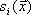the incident signal of the i-nth image. Assuming that the image function does not change between different observations and calling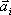the shifting vector, we can writewhere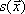is the reference image. It therefore follows that: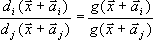indicating with the capital letter the (,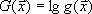), logarithm, we can write the preceding equation as follows:called: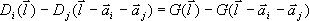Summing it up in all valid pixels so as to consider each couple once only, we minimize in comparisonwith the technique of square minimum the following function:In the end, through an iteration process, we obtain for each single pixel its related gain, which allows us to correct the images. In all images used for the application of this method (assuming they are produced by the same source) all regions with a high intensity gradient are masked (e.g. the solar edge and magnetic structures), so as to contain manipulations due to the numerical application of the method. At the end of the elaboration process, a calibrated image is provided, deprived of darkness and corrected for flat-field.